## Saturday, July 21, 2007

### Irodov Problem 1.37

This is a good problem to demonstrate the origin of centripetal acceleration. So I will solve it using two methods, one using only math and the other on by using centripetal acceleration.

Solution Using Centripetal Acceleration:As seen in the figure, the particle undergoes a tangential acceleration of a - this simply comes from the fact that the tangential velocity is given by v = at. In addition since the particle is turning around in a circle, it is also experience centripetal acceleration that is directed in the perpendicular (normal) direction towards the center of the circle and is equal to v2/r. The relation between the distance d traveled by the particle and the instantaneous tangential velocity is given by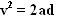, thus the centripetal acceleration is given by,. When the particle travels n fraction of the circle, the distance d covered is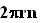. Thus, the centripetal acceleration of the particle is equal to. The total acceleration is thus given by,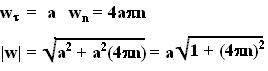Solution based on mathematics :
The instantaneous position of a particle rotating in a circle of radius r and a tangential velocity v
is given by,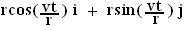Thus, for this problem the position vector of the particle is given by,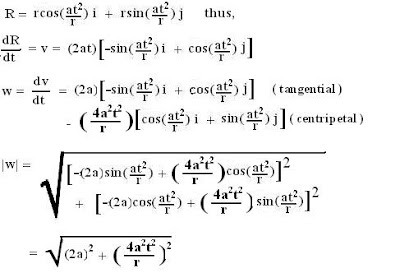Knowing that,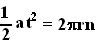we can reach the same result as obtain above. This solution shows the origin's of centripetal acceleration which comes from the basic fact that rotation itself by definition is acceleration!!!